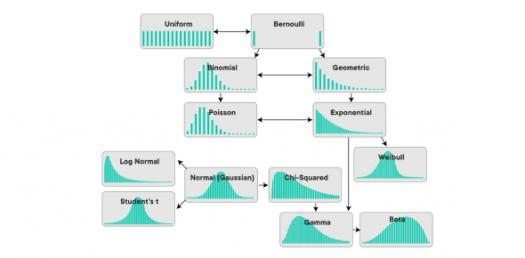# The Normal Distributions Test

10 Questions | Total Attempts: 899SettingsA normal distribution is a term in probability theory, which is a very common continuous probability distribution. Take this test to assess your knowledge of normal distribution.

• 1.
What is the area under a standard normal curve?
• A.

1

• B.

0

• C.

Not defined

• D.

Infinity

• 2.
Another name for normal distribution is...
• A.

Lagrangian distribution

• B.

Gaussian distribution

• C.

Laplacian distribution

• D.

Cauchy's distribution

• 3.
In a normal distribution, the mean, mode and median are all equal.
• A.

True

• B.

False

• C.

Depends on the type of normal distribution

• D.

Depends on some other factors

• 4.
On which of the following is the shape of a normal distribution curve dependent on?
• A.

Mean

• B.

Variance

• C.

Standard deviation

• D.

Mean deviation

• 5.
What is the value of the constant e in normal distribution?
• A.

2.4183

• B.

2.5183

• C.

2.6183

• D.

2.7183

• 6.
What is the value of mode in standard normal distribution?
• A.

1

• B.

0

• C.

Not fixed

• D.

None

• 7.
About which of the following is a normal distribution symmetric?
• A.

Covariance

• B.

Standard deviation

• C.

Mean

• D.

Variance

• 8.
The standard normal curve is symmetrical about the value of...
• A.

1

• B.

0

• C.

2

• D.

4

• 9.
What is the skewness of normal distribution?
• A.

1

• B.

Undefined

• C.

Positive

• D.

Negative

• 10.
What is the value of median in a standard normal distribution?
• A.

Infinity

• B.

Not fixed

• C.

1

• D.

0

Related TopicsBack to top